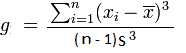## Skewness Calculator

 Result: Skewness Mean SD (Standard Deviation) Sum of (y(i)-y(mean)^3

The Skewness Calculator to calculate the asymmetry of the probability distribution of a real-valued random variable.

### Skewness Formulawhere:
x: Mean of samples
n: Total sample number
xi:The ith sample
g: Sample skewness
s: Standard Deviation of all samples

Thinkcalculator.com provides you helpful and handy calculator resources.